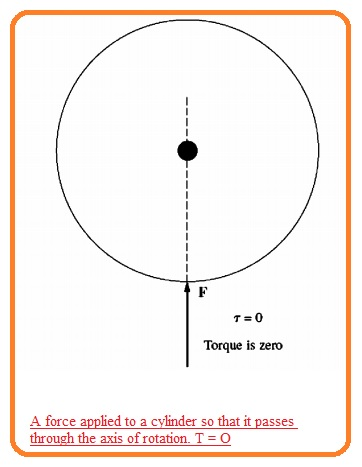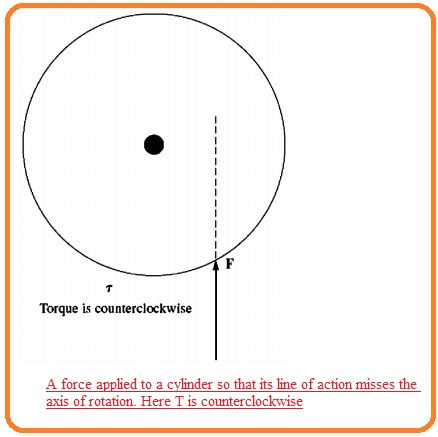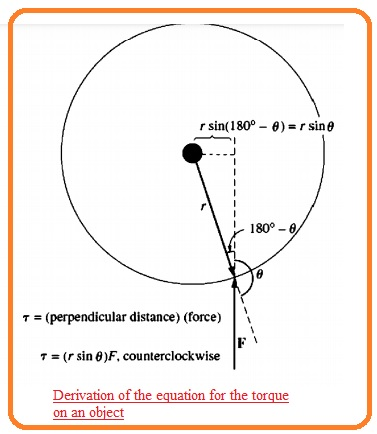Hi readers welcome to new post. In this post, we will have a detailed look at Rotational Motion, Newton’s Law, And Power Relationships. Each machine moves about its axis that known as the shaft of that machine. Since od rotational behavior of that machine, it is significant to have knowledge of rotational motion.

Here we cover details about the distance, acceleration, velocity and compare these terms with the newton law of motion. So let’s get started.

## What is Rotational Motion

• In normal there is a 3-dimensional vector is needed to discuss the rotation of any body. Though machines generally move at about shaft that is fixed due to this their rotation is bound at a single angular direction.
• With respect to the endpoint of the machine shaft, the direction of rotation will discuss in a clockwise and anticlockwise direction.
• The anticlockwise motor is taken as positive and clockwise as negative
• In case of movement about fix shaft all parameters taken as scalers.
• Let’s discuss each parameter related to rotational motion one by one.

What is Angular Position θ

• The angular position of any body is the angle over that it is directed calculated from a certain reference location.
• It is measured in radians and degrees and reslates to the linear behavior shown by distance in a straight line about.

What is Angular Velocity w

• The rate of change in angular position according to time is called angular velocity.
• It is taken as positive if rotation is anticlockwise.
• It is related to velocity in a straight line. Single dimensional linear velocity about straight line known as the rate of change of displacement along the line according to time.

V=dr/dt

• Like this angular velocity, w is explained as rate of change in angular displacement according to time.

w=dθ/dt

• The unit used for the angular position is radians then angular velocity will find in radians per second.
• In the case of normal electric machines technician generally used units other in place of radians/ seconds.
• Speed is measured in revolution/seconds or in per minute. Since speed is like significant parameters in the case of electrical machines it is necessary to employ numerous symbolic representations for speed do define in different units.
• Through the use of these symbolic representations, different problems can be decreases
• Wm is used to show angular velocity  in radians per second
• fm is used ot show  angular velocity  in revolutions/ second
• nm  used to show angular velocity defined in revolutions/minute

What is Angular Acceleration a

• The rate of change in angular velocity is known as angular acceleration.
•  It is positive if angular velocity is rising/ It is the rotational velocity about the parameter of acceleration at line.
• Single dimensions linear acceleration can express as.
• a=dv/dt
• angular acceleration is given as
• a=dw/dt

What is Torque

• For linear motion, the force given to the body results in velocity to vary in the non-existence of total force at body velocity has constant nature.
• The larger the force given to the body the velocity will vary.
• There is the same nature for rotation. If the body is moving angular velocity has constant till torque is existed.
• The larger the value of torque on the body there will be fast variation in the body
•  Torque twisting force on any body it can easily understand..
• Suppose that the cylinder shape body which is easy to rotate around its axis.
• If force provided to the cylinder in this technique in line of action moving though axis is shown in bellow figure.• In result, the cylinder will not show rotation.
• Though if a similar force is given in results the line of action of the force is moving at ninety degrees to the axis.
• rotate about its axis. If a force is applied to The cylinder in such a way that its line of axis can see here.• In a result, the cylinder will move in an anticlockwise direction. The torque at the cylinder relies on two factors first one is given force magnitude second one is the distance among the axis of the line of action of force.
• The torque exist on the body explained as the multiple of force at the body and least distance among the line of force and body axis of rotation
• If r is a vector directing from axis of rotation to the point where force is applied and if F is given force then torque is explained as.

t= (force applied)(perpendicular distance)
= (F) (r sin (θ)
= rF sin (θ)

• Here θ is the angle among the vector r and force F the direction of the torque is clockwise if it results in a clockwise movement and it is anticlockwise direction if rotation anticlockwise.• the unit of torque are Nm in case system international and pound-feet in English system

### What is Newton’s Law of Rotation

• Newton’s law for different bodies that are moving in a straight line discusses the relationship between the force given to body and acceleration produced due to force..
• The equation is given here
• F=ma
• F is  force given to the body
• m is mass of the body
• a is acceleration
• in case of system, international units force unit is newton mass unit is KG and acceleration nit is m/second square.
• in case of English system, force is calculated in pounds, in this system mass unit is a slug and the acceleration unit is feet/ second square
• the single equation explains the relationships among the torque given at the body and acceleration.
• This connection is known as newton’s law of rotation and mention here.

t = Ja

• Here t is the total torque applied in units of newton meter and a is angular acceleration in units of radians per seconds square.
• A similar operation relies for a body in case if linear motion.
• It is known as movement of inertia of the body.

That is all about Rotational Motion, Newton’s Law, And Power Relationships if you has any query ask in the comments. Thanks for reading have a nice day.